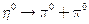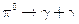Chapter 33, Problem 27PE

Chapter
Section
Textbook Problem

One decay mode for the eta−zero meson is(a) Write the decay in terms at the quark constituents.(b) How much energy is released?(c) What is the ultimate release of energy, given the decay mode for the pi zero isTo determine

The decay mode η0π0+π0 in terms of quark structure.

Explanation

Given:

Given reaction is, η0π0+π0

Calculation:

Quark structure of η0=uu¯+dd¯

Quark structure of π0=uu¯+dd¯

Therefore, the equation in terms of quarks is given by,

η0π0+π0

uu¯+dd¯

To determine

(b)

The energy released in the decay of η0 particle given by η0π0+π0

To determine

(c)

The ultimate release of energy if the decay product π0 of the reaction η0π0+π0 is again

decayed by π0γ+γ

Still sussing out bartleby?

Check out a sample textbook solution.

See a sample solution

The Solution to Your Study Problems

Bartleby provides explanations to thousands of textbook problems written by our experts, many with advanced degrees!

Get Started

Find more solutions based on key concepts